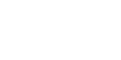Construction:
1) Find the direction of the thread at an angle of 45°. We estimated the bend.
2) Perpendicular to the fold draw a line where the delay amount (2) equal to the midpoint Smad, plus allowances for processing.
A1A = BB1 = 7 - 10 cm А1Б1 - mid Smad
3) From point A1 at angle of 15 gr. hold the line, which pushes the value of the left-Smad (1) k (5) + 8 cm А1Г - left shoulder Smad
4) From the point B1 is also at an angle of 15°., hold the line, which pushes the value of the right shoulder (3) with k (2,5).
5) At the expected bend from the perpendicular lay off the depth of the Smad (5) with k (2) DD1 = value (5) (Smad depth) x k (2)
6) Through the points B - D1 - G conduct an arc equal to the lower of the SAG (4) obtained by the measurement, plus 5-10 cm for processing
ГД1В = value (4) + 5 (10) cm - lower slack Smad